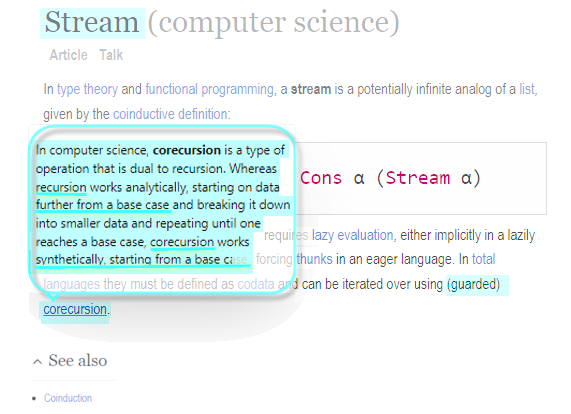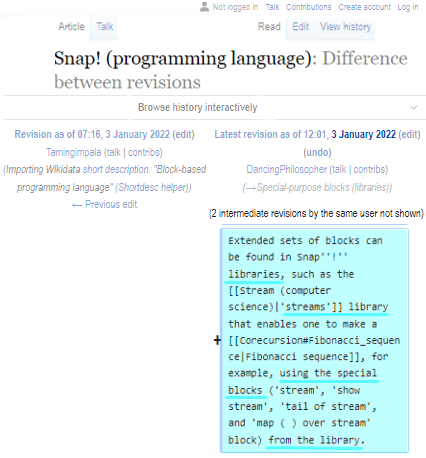# Fibonacci stream

:~D

Isn't it awesome that you can do that?

Despite being a non-math and a non-CS person, I nevertheless understand and appreciate

... this particular ( (advanced) CS ) thingy...

...otherwise known (by CS experts, not me) as 'stream'...

too.

Wow, this doesn't happen every day.

So thank you, warped_wart_wars, for this gift.

P. S.

whatI found.

As I haven't yet heard of corecursion, I clicked on it and in the article I read that "many functions - traditionally analyzed as recursive - can be more naturally interpreted as corecursive functions(...)"

"Corecursion creates (potentially infinite) codata, whereas ordinary recursion analyzes (necessarily finite) data."

Also, it says that "the Fibonacci sequence is a recurrence relation of order 2".

That's all new to me. I bet Brian could explain it using examples from Snap!.

The name "corecursion" is new to me, too!

As for recurrence relations, suppose I show you this table:

1    1
2    4
3    9
4   16


and ask you what's going on in it. The standard, functional way to look at it is to look across each row, and say "the second number is the square of the first." But another way is to look down the second column. (The first column is trivial.) What are the differences between successive values? 4-1=3; 9-4=5; 16-9=7. These are successive odd numbers. So I'm going to guess that the next value is 16+9=25, and the one after that is 25+11=36. In this way I can fill in the table without knowing that the values are related to the consecutive integers by a common function.

For that table, the functional approach works at a glance, and there's no reason to look for a recurrence relation. But suppose the table were

1     6
2     4
3     4
4     6
5    10
6    16


Now it's not obvious whether the numbers in the second column are any polynomial function of the numbers in the first column. So we'll try computing differences:

1     6
2     4    -2
3     4     0
4     6     2
5    10     4
6    16     6


The differences aren't constant. But look what happens if we take their differences:

1     6
2     4    -2
3     4     0    2
4     6     2    2
5    10     4    2
6    16     6    2


Aha! Constant second differences. Now we can fill in more differences:

1     6
2     4    -2
3     4     0    2
4     6     2    2
5    10     4    2
6    16     6    2
8
10
12


and we can use those to compute the desired result values:

1     6
2     4    -2
3     4     0    2
4     6     2    2
5    10     4    2
6    16     6    2
24     8
34    10
46    12


This technique lets us compute the desired results without reference to the first column, the underlying independent variable. (This function is actually f(x)=x²−5x+10.)

I would have taken a while to figure that out if you hadn't said it, because I just like figuring these things out.

Sorry. :~)

Can you think of another one?

No, I meant you make a table and I guess what function it is.

This is actually basic calculus (correct me if I'm wrong). The first derivative of $$f(x)$$ (written as $$f'(x)$$ is $$2x-5$$, and the second derivative ($$f'(x)$$) is just $$2$$. You can see this in the table how the first column of differences isn't constant, but how the second column is.
If you don't know what derivatives are, derivatives basically measure rate of change, or slope, but "at a certain point in time."

That's what those RANDOM blocks are for! They make a table and you have to figure out what random values it used.

Yes. Technically it's not the derivative because it's not the slope at a single point; it's called "finite differences." So you don't have to know calculus to learn recurrence relations (at least not for polynomial functions), and in fact finite differences are a way into calculus pedagogically.

I like it when you say "Aha!" as if I am witnessing (and/or being part of) a discovery (and/or creative) process as opposed to reading a boring textbook or standard wikipedia article about math, where everything is presented as being totally unsurprising & well-established (for a thousand years); or 'self-evident', which is driving lay readers away.

I remember having some calculus in highschool, but I don't remember it being introduced via finite differences.
I don't even remember hearing the term itself.

P. S.

I have not looked it up in wikipedia; I'd rather have you answer the following question; namely, do calculation of f.d. need to have integers only as inputs. It may sound trivial, but I am asking it anyway.

I meant that it was a related concept. Also I just found out there's "calculus with finite differences."

No. It's just that the arithmetic is easier with integers.

So I'm not the only one who has not heard that what used to be called recursion has actually been split into two categories:
a) the recursion in the narrower sense of the word one one hand, and
b) the corecursion, on the other hand.